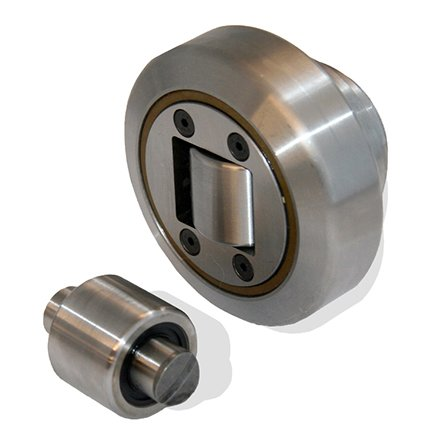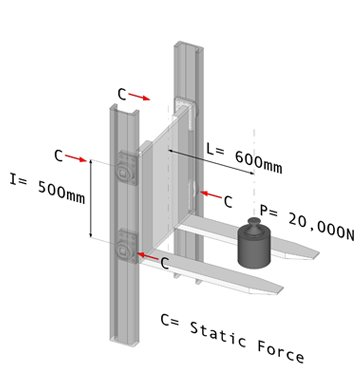# WORKED EXAMPLE - CALCULATING HERTZIAN PRESSURE## A 2 tonne load is placed 600mm from the point of suspension. The bearings are spaced 500mm apart. Which bearings should be used?

Using the Moment-Balance formula: C = (P . L) / (2 . I)

where:
P = The load being moved (N)
L = Distance between the load (P) and the suspension point (mm)
I = Distance between the bearings (mm)
C = Maximum allowable static force per bearing (N)

Assuming the bottom pair of bearings are level with the point of suspension then I = 500mm. The load is cantilevered out at 600mm and hence this is the value of L. Here P is 20000N.

Substituting these numbers into the equation gives
C = 12000N

Looking up this value of C on the Hertzian Pressure table shows that 4.058 is the required bearing size.

If you need help with any of these calculations, call Kate or Matt (01908 511733) or email a drawing to: sales@euro-bearings.com